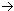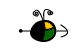Energy Change for a Nuclear Rection

During nuclear changes, either some mass is converted into energy or some energy is converted into mass.  Which occurs is dependent upon the specifics of the individual reaction.  From this change in mass we can calculate its energy equivalent using Einstein's equation, E = mc2.

Determining the Energy Change of a Nuclear Reaction

To find the energy change for a nuclear reaction you must know the masses of each species in the equation for the reaction.  To calculate the energy change for a nuclear reaction:

• Calculate the sum of the masses of all of the products, and the sum of the masses of all the reactants,
• Calculate the change in mass by subtracting the combined mass of the reactants from the combined mass of the products,
• Convert the change in mass into its equivalent change in energy using Einstein's equation.
• Convert the energy change from J/atom to kJ/mol of atoms.
Example:  Calculate the energy change for the following nuclear reaction.  The masses of each species are given below.

1 neutron + 23589Rb + 144Ce + 3 electrons + 3 neutrons

Masses:
neutron = 1.00867 amu
electron = 0.00055 amu
uranium-235 = 234.9934 amu
rubidium-89 = 88.8913 amu
cerium-144 = 143.8817 amu

• Calculate the combined masses of the products and of the reactants.
Mass of Products = 88.8913 amu + 143.8817 amu + 3 (0.00055 amu) + 3 (1.00867 amu) = 235.8007 amu
Mass of Reactants = 1.00867 amu + 234.9934 amu = 236.0021 amu
• Calculate the change in mass for the reaction (mass of products - mass of reactants).
Dmass = 235.8007 amu - 236.0021 amu = - 0.2014 amu
• Convert the change in mass into energy using Einstein's equation.  Remember to change the mass into kilograms.
DE = (- 0.2014 amu)(1.6606 x 10-27 kg/amu)(2.9979 x 108 m/s)2 = - 3.006 x 10-11 J
• Convert the energy change per atom of uranium-235 into kJ/mol of uranium-235.
(- 3.006 x 10-11 J/atom)(1 kJ/1000 J)(6.023 x 1023 atoms/mol) = - 1.811 x 1010 kJ/mol of U-235